# High School Math : Simplifying Polynomial Functions

## Example Questions

### Example Question #1 : Polynomials

Simplify the following polynomial: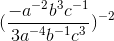Possible Answers: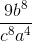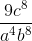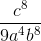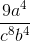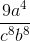Correct answer:Explanation:

To simplify the polynomial, begin by combining like terms: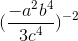### Example Question #21 : Pre Calculus

Simplify the following polynomial function: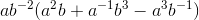Possible Answers: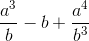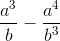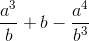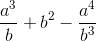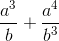Correct answer:Explanation:

First, multiply the outside term with each term within the parentheses: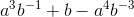Rearranging the polynomial into fractional form, we get:### All High School Math Resources